# filt2block

## Syntax

``filt2block(b)``
``filt2block(b,'subsystem')``
``filt2block(___,'FilterStructure',structure)``
``filt2block(b,a)``
``filt2block(b,a,'subsystem')``
``filt2block(___,'FilterStructure',structure)``
``filt2block(sos)``
``filt2block(sos,'subsystem')``
``filt2block(___,'FilterStructure',structure)``
``filt2block(d)``
``filt2block(d,'subsystem')``
``filt2block(___,'FilterStructure',structure)``
``filt2block(___,Name,Value)``

## Description

example

````filt2block(b)` generates a `Discrete FIR Filter block ` with filter coefficients, `b`.```
````filt2block(b,'subsystem')` generates a Simulink® subsystem block that implements an FIR filter using sum, gain, and delay blocks.```

example

````filt2block(___,'FilterStructure',structure)` specifies the filter `structure` for the FIR filter.```

example

````filt2block(b,a)` generates a `Discrete Filter block` with numerator coefficients, `b`, and denominator coefficients, `a`.```
````filt2block(b,a,'subsystem')` generates a Simulink subsystem block that implements an IIR filter using sum, gain, and delay blocks.```

example

````filt2block(___,'FilterStructure',structure)` specifies the filter `structure` for the IIR filter.```
````filt2block(sos)` generates a `Biquad Filter block` with second order sections matrix, `sos`. `sos` is a K-by-6 matrix, where the number of sections, `K`, must be greater than or equal to 2. You must have the DSP System Toolbox™ software installed to use this syntax.```

example

````filt2block(sos,'subsystem')` generates a Simulink subsystem block that implements a biquad filter using sum, gain, and delay blocks.```
````filt2block(___,'FilterStructure',structure)` specifies the filter `structure` for the biquad filter.```
````filt2block(d)` generates a Simulink block that implements a digital filter, `d`. Use the function `designfilt` to create `d`. The block is a ```Discrete FIR Filter block``` if `d` is FIR and a ```Biquad Filter block``` if `d` is IIR.```
````filt2block(d,'subsystem')` generates a Simulink subsystem block that implements `d` using sum, gain, and delay blocks.```

example

````filt2block(___,'FilterStructure',structure)` specifies the filter `structure` to implement `d`.```

example

````filt2block(___,Name,Value)` uses additional options specified by one or more `Name,Value` pair arguments.```

## Examples

collapse all

Design a 30th-order FIR filter using the window method. Specify a cutoff frequency of π/4 rad/sample. Create a Simulink® block.

```b = fir1(30,0.25); filt2block(b) ```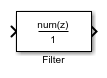Design a 30th-order IIR Butterworth filter. Specify a cutoff frequency of π/4 rad/sample. Create a Simulink® block.

```[b,a] = butter(30,0.25); filt2block(b,a) ```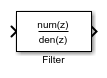Design a 30th-order FIR filter using the window method. Specify a cutoff frequency of π/4 rad/sample. Create a Simulink® block with a direct form I transposed structure.

```b = fir1(30,0.25); filt2block(b,'FilterStructure','directFormTransposed') ```Design a 30th-order IIR Butterworth filter. Specify a cutoff frequency of π/4 rad/sample. Create a Simulink® block with a direct form I structure.

```[b,a] = butter(30,0.25); filt2block(b,a,'FilterStructure','directForm1') ```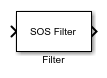Design a 5-th order Butterworth filter with a cutoff frequency of π/5 rad/sample. Obtain the filter in biquad form and generate a Simulink® subsystem block from the second order sections.

```[z,p,k] = butter(5,0.2); sos = zp2sos(z,p,k); filt2block(sos,'subsystem') ```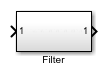Generate a Simulink® subsystem block that implements an FIR lowpass filter using sum, gain, and delay blocks. Specify the input processing to be elements as channels by specifying `'FrameBasedProcessing'` as `false`.

```B = fir1(30,.25); filt2block(B,'subsystem','BlockName','Lowpass FIR',... 'FrameBasedProcessing',false) ```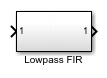Design a highpass elliptic filter with normalized stopband frequency 0.45 and normalized passband frequency 0.55. Specify a stopband attenuation of 40Design a highpass elliptic filter with normalized stopband frequency 0.45 and normalized passband frequency 0.55. Specify a stopband attenuation of 40 dB and a passband ripple of 0.5 dB. Implement the filter as a Direct Form II structure, call it "HP", and place it in a new Simulink® model.

```d = designfilt('highpassiir','DesignMethod','ellip', ... 'StopbandFrequency',0.45,'PassbandFrequency',0.55, ... 'StopbandAttenuation',40,'PassbandRipple',0.5); filt2block(d,'subsystem','FilterStructure','directForm2', ... 'Destination','new','BlockName','HP') ```## Input Arguments

collapse all

Numerator filter coefficients, specified as a row or column vector. The filter coefficients are ordered in descending powers of z–1 with the first element corresponding to the coefficient for z0.

Example: `b = fir1(30,0.25);`

Data Types: `single` | `double`
Complex Number Support: Yes

Denominator filter coefficients, specified as a row or column vector. The filter coefficients are ordered in descending powers of z–1 with the first element corresponding to the coefficient for z0. The first filter coefficient must be 1.

Data Types: `single` | `double`
Complex Number Support: Yes

Second order section matrix, specified as a K-by-2 matrix. Each row of the matrix contains the coefficients for a biquadratic rational function in z–1. The Z-transform of the Kth rational biquadratic system impulse response is

`${H}_{k}\left(z\right)=\frac{{B}_{k}\left(1\right)+{B}_{k}\left(2\right){z}^{-1}+{B}_{k}\left(3\right){z}^{-2}}{{A}_{k}\left(1\right)+{A}_{k}\left(2\right){z}^{-1}+{A}_{k}\left(3\right){z}^{-2}}$`

The coefficients in the Kth row of the matrix, `sos`, are ordered as follows:

`$\left[\begin{array}{cccccc}{B}_{k}\left(1\right)& {B}_{k}\left(2\right)& {B}_{k}\left(3\right)& {A}_{k}\left(1\right)& {A}_{k}\left(2\right)& {A}_{k}\left(3\right)\end{array}\right]$`

The frequency response of the filter is its transfer function evaluated on the unit circle with z = ej2πf.

Data Types: `single` | `double`
Complex Number Support: Yes

Digital filter, specified as a `digitalFilter` object. Use `designfilt` to generate a digital filter based on frequency-response specifications.

Example: ```d = designfilt('lowpassiir','FilterOrder',3,'HalfPowerFrequency',0.5)``` specifies a third-order Butterworth filter with normalized 3 dB frequency 0.5π rad/sample.

Filter structure, specified as a character vector or string scalar. Valid options for `structure` depend on the input arguments. The following table lists the valid filter structures by input.

InputFilter Structures
`b``'directForm'` (default), `'directFormTransposed'`, `'directFormSymmetric'`, `'directFormAntiSymmetric'`, `'overlapAdd'`. The `'overlapAdd'` structure is only available when you omit `'subsystem'` and requires a DSP System Toolbox software license.
`a``'directForm2'` (default), `'directForm1'`, `'directForm1Transposed'`, `'directForm2'`, `'directForm2Transposed'`
`sos``'directForm2Transposed'` (default), `'directForm1'`, `'directForm1Transposed'`, `'directForm2'`
`d`
• For FIR filters: `'directForm'` (default), `'directFormTransposed'`, `'directFormSymmetric'`, `'directFormAntiSymmetric'`, `'overlapAdd'`. The `'overlapAdd'` structure is only available when you omit `'subsystem'` and requires a DSP System Toolbox software license.

• For IIR filters: `'directForm2Transposed'` (default), `'directForm1'`, `'directForm1Transposed'`, `'directForm2'`

### Name-Value Arguments

Specify optional pairs of arguments as `Name1=Value1,...,NameN=ValueN`, where `Name` is the argument name and `Value` is the corresponding value. Name-value arguments must appear after other arguments, but the order of the pairs does not matter.

Before R2021a, use commas to separate each name and value, and enclose `Name` in quotes.

Example: ```filt2block(...,'subsystem','BlockName','Lowpass FIR','FrameBasedProcessing',false)```

Destination for the Simulink filter block, specified as a character vector or string scalar. You can add the filter block to your current model with `'current'`, add the filter block to a new model with `'new'`, or specify the name of an existing model.

Example: ```filt2block([1 2 1],'Destination','MyModel','BlockName','New block')```

Data Types: `char` | `string`

Block name, specified as a character vector or string scalar.

Data Types: `char` | `string`

Overwrite block, specified as a logical `false` or `true`. If you use a value for `'BlockName'` that is the same as an existing block, the value of `'OverwriteBlock'` determines whether the block is overwritten. The default value is `false`.

Data Types: `logical`

Map coefficients to ports, specified as a logical `false` or `true`.

Data Types: `logical`

Coefficient variable names, specified as a cell array of character vectors or a string array. This name-value pair is only applicable when `'MapCoefficientsToPorts'` is `true`. The default values are `{'Num'}`, `{'Num','Den'}`, and `{'Num','Den','g'}` for FIR, IIR, and biquad filters.

Data Types: `cell` | `string`

Frame-based or sample-based processing, specified as a logical `true` or `false`. The default is `true` and frame-based processing is used.

Data Types: `logical`

Remove zero-gain blocks, specified as a logical `true` or `false`. By default zero-gain blocks are removed.

Data Types: `logical`

Replace unity-gain blocks with direct connection, specified as a logical `true` or `false`. The default is `true`.

Data Types: `logical`

Replace negative unity-gain blocks with a sign change at the nearest block, specified as a logical `true` or `false`. The default is `true`.

Data Types: `logical`

Replace cascaded delays with a single delay, specified as a logical `true` or `false`. The default is `true`.

Data Types: `logical`

## Version History

Introduced in R2013a